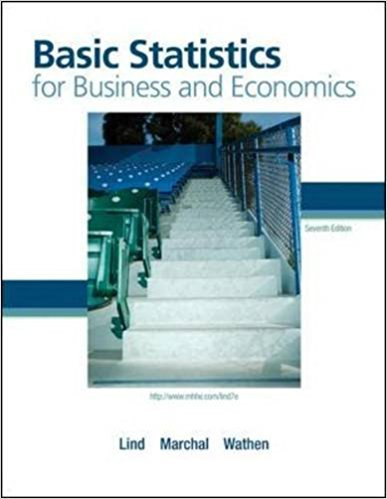×
Get Full Access to Basic Statistics For Business And Economics - 7 Edition - Chapter 3 - Problem 32e
Get Full Access to Basic Statistics For Business And Economics - 7 Edition - Chapter 3 - Problem 32e

×

# A sample of the personnel files of eight employees at theISBN: 9780077384470 64

## Solution for problem 32E Chapter 3

Basic Statistics for Business and Economics | 7th Edition

• Textbook Solutions
• 2901 Step-by-step solutions solved by professors and subject experts
• Get 24/7 help from StudySoup virtual teaching assistantsBasic Statistics for Business and Economics | 7th Edition

4 5 1 410 Reviews
29
0
Problem 32E

Problem 32E

A sample of the personnel files of eight employees at the Pawnee location of Acme Carpet Cleaners, Inc., revealed that during the last six-month period they lost the following number of days due to illness:

 2 0 6 3 10 4 1 2

A sample of eight employees during the same period at the Chickpee location of Acme Carpets revealed they lost the following number of days due to illness.

 2 0 1 0 5 0 1 0

As the director of human relations, compare the two locations. What would you recommend?

Step-by-Step Solution:

Solution :

Step 1 of 1:

Our goal is :

We need to find compare the two locations.

Given 8 employees at the Pawnee location of Acme Carpet Cleaners.

So n=8.

Consider the data is

2, 0, 6, 3, 10, 4, 1, 2.

Now we have to calculate range.

First we have to arrange in ascending order.

0, 1, 2, 2, 3, 4, 6, 10.

Here the maximum value = 10 and the minimum value = 0.

The range formula is

Range = Maximum value-Minimum value

Range = 10-0

Range = 10

Therefore, the range is 10.

First we have to arrange in ascending order.

0, 1, 2, 2, 3, 4, 6, 10.

Then we take the 2 middle 2 and 3 values.

Now we have to add the 2 values and divide by 2.

Median =Median =Median = 2.5

Therefore, the median is 2.5.

Now we have to find the mean.

The mean formula is

Mean () =Mean =Mean =Mean = 3.5

Therefore, the mean is 3.5.

Given 8 employees during the same period at the Chickpea location.

So n=8.

Consider the data is

2, 0, 1, 0, 5, 0, 1, 0.

Now we have to calculate range.

First we have to arrange in ascending order.

0, 1, 2, 2, 3, 4, 6, 10.

Here the maximum value = 10 and the minimum value = 0.

The range formula is

Range = Maximum value-Minimum value

Range = 5-0

Range = 5

Therefore, the range is 5.

First we have to arrange in ascending order.

0, 0, 0, 0, 1, 1, 2, 5.

Then we take the 2 middle 0 and 1 values.

Now we have to add the 2 values and divide by 2.

Median =Median =Median = 0.5

Therefore, the median is 0.5.

Now we have to find the mean.

The mean formula is

Mean () =Mean =Mean =Mean = 1.125

Therefore, the mean is 1.125.

The Pawnee and Chickpea table is given below.

 Location Range Median Mean Pawnee 10 2.5 3.5 Chickpea 5 0.5 1.25

Therefore, the mean and median location were higher, but there was also a more variation in Pawnee.

Step 2 of 1

##### ISBN: 9780077384470

Since the solution to 32E from 3 chapter was answered, more than 2261 students have viewed the full step-by-step answer. The full step-by-step solution to problem: 32E from chapter: 3 was answered by , our top Business solution expert on 08/23/17, 08:36AM. This full solution covers the following key subjects: acme, sample, Revealed, period, Lost. This expansive textbook survival guide covers 6 chapters, and 325 solutions. The answer to “A sample of the personnel files of eight employees at the Pawnee location of Acme Carpet Cleaners, Inc., revealed that during the last six-month period they lost the following number of days due to illness:206310412A sample of eight employees during the same period at the Chickpee location of Acme Carpets revealed they lost the following number of days due to illness.20105010As the director of human relations, compare the two locations. What would you recommend?” is broken down into a number of easy to follow steps, and 74 words. This textbook survival guide was created for the textbook: Basic Statistics for Business and Economics , edition: 7. Basic Statistics for Business and Economics was written by and is associated to the ISBN: 9780077384470.

Unlock Textbook Solution